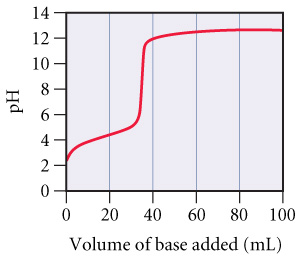Chemistry Practice Problems Acid and Base Titration Curves Practice Problems Solution: A 0.446-g sample of an unknown monoprotic acid was...

⚠️Our tutors found the solution shown to be helpful for the problem you're searching for. We don't have the exact solution yet.

# Solution: A 0.446-g sample of an unknown monoprotic acid was titrated with 0.105 M KOH and the resulting titration curve is shown in the figure.Determine pKa of the acid.

###### Problem

A 0.446-g sample of an unknown monoprotic acid was titrated with 0.105 M KOH and the resulting titration curve is shown in the figure.Determine pKa of the acid.

Acid and Base Titration Curves

Acid and Base Titration Curves

#### Q. Consider the following curve for the titration of a weak base with a strong acid and answer each of the following questions.At what volume of added ac...

Solved • Thu Jan 03 2019 09:31:13 GMT-0500 (EST)

Acid and Base Titration Curves

#### Q. A weak unknown monoprotic acid is titrated with a strong base. The titration curve is shown below.Find Ka for the unknown acid.

Solved • Thu Jan 03 2019 09:30:31 GMT-0500 (EST)

Acid and Base Titration Curves

#### Q. Consider the following titration curves for two weak bases, both titrated with 0.100 M HCl.Which of the two bases has the larger Kb?

Solved • Thu Jan 03 2019 09:30:22 GMT-0500 (EST)

Acid and Base Titration Curves

#### Q. A 0.229-g sample of an unknown monoprotic acid was titrated with 0.112 M NaOH and the resulting titration curve is shown in the figure.Determine pKa o...

Solved • Thu Jan 03 2019 09:30:22 GMT-0500 (EST)